American Journal of Theoretical and Applied Statistics
Volume 4, Issue 4, July 2015, Pages: 300-304

Application of Response Surface Methodology for Optimization of Potato Tuber Yield

Dennis Kariuki Muriithi

Faculty of Business Studies, Chuka University, Chuka, KenyaDennis Kariuki Muriithi. Application of Response Surface Methodology for Optimization of Potato Tuber Yield. American Journal of Theoretical and Applied Statistics. Vol. 4, No. 4, 2015, pp. 300-304. doi: 10.11648/j.ajtas.20150404.20

Abstract: The Author investigates the operating conditions required for optimal production of potato tuber yield in Kenya. This will help potato farmers to safe extra cost of input in potato farming. The potato production process was optimized by the application of factorial design 23 and response surface methodology. The combined effects of water, Nitrogen and Phosphorus mineral nutrients were investigated and optimized using response surface methodology. It was found that the optimum production conditions for the potato tuber yield were 70.04% irrigation water, 124.75Kg/Ha of Nitrogen supplied as urea and 191.04Kg/Ha phosphorus supplied as triple super phosphate. At the optimum condition one can reach to a potato tuber yield of 19.36Kg/plot of 1.8meters by 2.25 meters. Increased productivity of potatoes can improve the livelihood of smallholder potato farmers in Kenya and safe the farmers extra cost of input. Finally, i hope that the approach applied in this study of potatoes can be useful for research on other commodities, leading to a better understanding of overall crop production.

Keywords: Response Surface Methodology, Potato, Nitrogen, Phosphorus, Factorial Design, Experiment, Optimization, Yield

1. Introduction

Response surface methodology is a collection of statistical and mathematical techniques useful for developing, improving, and optimizing processes Myers . It also has important applications in the design, development, and formulation of new products, as well as in the improvement of existing product designs. For instance, the growth of a plant is affected by a certain amount of water x1 and sunshine x2. The plant can grow under any combination of treatment x1 and x2. Therefore, water and sunshine can vary continuously. When treatments are from a continuous range of values, then a Response Surface Methodology is useful for developing, improving, and optimizing the response variable. In this case, the plant growth y is the response variable, and it is a function of water and sunshine. It can be expressed as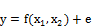(1)

The variables x1 and x2 are predictor variables where the response y depends on them. The dependent variable y is a function of x1, x2 and the experimental error term, denoted as e. The error term e represents any measurement error on the response, as well as other type of variations not counted in the function. It is a statistical error that is assumed to distribute normally with zero mean and variance. In most Response surface methodology problems, the true response function f is unknown. In order to get the most efficient result in the approximation of polynomials the proper experimental design must be used to collect data. Once the data are collected, the Method of Least Square is used to estimate the parameters in the polynomials, G.E.P. Box and Hunter . The response surface analysis is performed by using the fitted surface. The response surface designs are types of designs for fitting response surface. The objective of this study is to determine the optimal operating condition for the system, required for optimal potato tuber yield's using Response Surface Methodology. The study has taken into consideration the fact that the growth, development, and consequently yield of crops are highly influenced by available soil moisture, Alem . Therefore, irrigation water, Nitrogen and Phosphorus mineral nutrient influence potato production according to K. Gathungu .

2. Material and Methods

2.1. Factorial Experimental Design

Factorial designs are widely used in experiments involving several factors where it is necessary to investigate the joint effects of the factors on a response variable. Such an experiment allows the investigator to study the effect of each factor on the response variable, as well as the effects of interactions between factors on the response variable, Myers . A very important special case of the factorial design is that where each of the k factors of interest has only two levels. Because each replicate of such a design has exactly 2k experimental trials, it is called 2k factorial designs. The simplest factorial experiment contains two levels for each of two factors. Suppose an engineer wishes to study the total power used by each of two different motors, P and Q, running at each of two different speeds, 2000 or 3000RPM (revolution per minutes). The factorial experiment would consist of four experimental units: motor P at 2000RPM, motor Q at 2000RPM, motor P at 3000RPM, and motor Q at 3000RPM. Each combination of a single level selected from every factor is present once. In this paper the researcher restricted himself to three factors, Namely; Water, Nitrogen and Phosphorus mineral nutrient. The three were chosen as predictors’ variable and potato tuber yield was the dependent variable. The coded values of the variables were determined by the following equation.where xi is a coded variable of the ith variable, X0 is average value of variable in high and low levels, X is (variable at high level - variable at low level)/2 and Xi is a encoded value of the ith test variable. The factorial point is defined as ±1 unit for each factor. The Codes, ranges and levels of independent variables of Water, Nitrogen and Phosphorus mineral nutrient are as given in Table 1.

Table 1: Codes, ranges and levels of independent variables of Water, Nitrogen and Phosphorus

 Symbols Predictor variable Coded levels -1 0 1 x1 Water (Irrigation rate) 40% 70% 100% x2 Nitrogen (Urea 46% N) 75kg/ha 112.5kg/ha 150kg/ha x3 Phosphorus (46% P2O5 115 kg/ha 172.5kg/ha 230kg/ha

Scientists from Chuka University design and conducted an experiment where they planted Potatoes in a rain shelter at the Horticultural Research and Teaching Farm of Egerton University, Njoro. This forms the source of the data that was used in this study.

2.2. Response Surface Method

In most problem the form of the relationship between response and predictor variable is unknown. Thus the first step in Response surface methodology is to find a suitable approximation for the true functional relationship between y and the set of predictors variables usually a low-order polynomial in some region of the predictor variable is employed. The approximating model is based on observed data from the process. According to Montgomery , Muriithi  and Myers , multiple regression is collection of statistical techniques useful for building the types of models required in Response surface methodology. In this case, a response is well modelled by a linear relationship of predictor variables of first order model provided in Equation 2.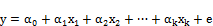(2)

where y is dependent variable,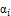;i=0,1,...,k is the regression coefficients that measure the expected change in the response y per unit change xk when other Predictor variables are held constant.

When the experimenter is relatively close to the optimum, a model that incorporates curvature is usually requires to approximate the response. In this case a polynomial of higher degree must be used such as second-order model provided in equation 3.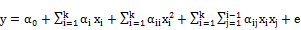(3)

where y is predicted response,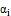,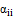andare the regression coefficients, they represent the linear, Quadratic and Cubical effect of predicted variable on response. Using equation 3, the researcher opts to find the optimum set of operating conditions for the x’s and the nature of response surface.

Details of experimental designs for fitting response surfaces are found in Montgomery  and Khuri.To determine the effect of treatment, data was analyzed using Analysis of Variance. P-value of less than 5% were regard as statistically significant. Data was analyzed using R- Program (A statistical software).

3. Empirical Results and Discussion

3.1. Fitting a Regression Model for Potato Tuber Yield

Experimental potato tuber yields were analyzed to get a regression model. The estimated coefficients of the regression model are given in Table 2. The large value of the coefficient of multiple determination (R2 = 0.9741) reveals that the model adequately represents the experimental results. This section represents result of the regression model.

Table 2: Estimates Coefficients of the Regression model.

 Variables Estimates Std. Error t-Value pr (>| t |) Intercept 19.3074 0.3471 46.976 < 2e-16 x1 0.23567 0.1607 14.665 4.43E-11 x2 0.19289 0.1607 12.003 1.00E-09 x3 0.16567 0.1607 10.309 9.89E-09 x12 -3.3989 0.2783 -12.212 7.70E-10 x22 -0.4822 0.2783 -1.733 0.10128 x32 -0.4589 .0.2783 -1.649 0.11756 x1x2 -0.3383 0.1968 -1.719 0.10376 x1x3 -0.735 0.1968 -3.735 0.00165 x2x3 0.3617 0.1968 1.838 0.04366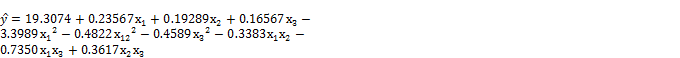(4)

Table 2, shows the regression estimates, standard error of estimate, t-value and Probability value associated with estimate of linear, quadratic and interaction effects. Employing a 5% criterion of statistical significance, x1, x2 and x3 factors had significant effect on potato tuber yield. It was found that factors main effect had a significant effect on potato tuber yields. For instance, an increase of x2 by one unit, the yield of potato would increase by 0.1929 units. Indeed, an increase of x3 by one unit, the yield of potato would increase by 0.1657 units. Similarly, an increase of x1 by one unit, the yield of potato would increase by 0.236 units. The study revealed that the interaction between x2 x3 and x1x3 were statistically significant on potato tuber yield, all reporting a probability value of less than 0.05 (0.044<0.05 and 0.0012<0.05 respectively). This implies that x2 and x3 were very critical in production of potatoes by potato farmers in Kenya. In addition, the results shows a coefficient of determination of 97.41% (R2 = 0.9741). This implies that 97.41% of variation in the model can be accounted for by the variables (x1, x2 and x3). However, it is important to determine the required level of the three factors that can guarantee the farmer maximum potato tuber yield without incurring extra cost of input.

Table 3: Analysis of Variance

 Df Sum Squares mean Squares F-value pr (>F ) Model 9 297.43 33.048 71.17 1.02E-11 Residuals 17 7.902 0.465 Total 26 305.332

Table 3, shows the overall effect of the regression model. It was found that the regression model was highly statistically significant in assessing the effect of x1, x2 and x3 on production of potatoes in Kenya. The overall p-value of the model was 0.0000000000102<0.05. This study indicates that the model can be considered statistically significant according to the F-test with 95% of confidence, as the F-value of 71.17 is much higher than F (9,17)=2.4943, showing that the model adequately represents the experimental results.

3.2. Response Surface Analysis

In this study it was important to determine the required level of the factors that can guarantee the farmer maximum potato tuber yield without incurring extra cost of input and the nature of the optimal value that is; maximum, minimum or saddle point. Response Surface Methodology can be illustrated with three-dimensional plots by presenting the response in function of two factors and keeping the other constant. It is visualized by the yield of potato tuber in relation to the water, Nitrogen and Phosphorus mineral nutrient in Figure 1, 2 and 3.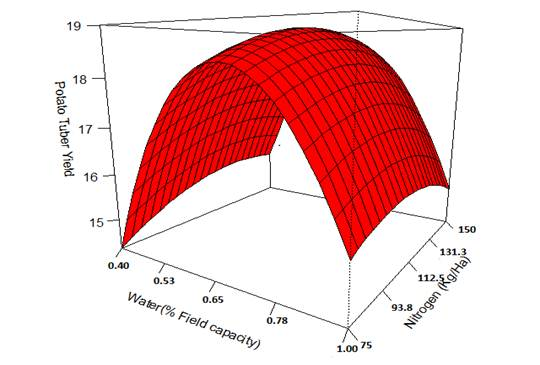Figure 1: Potato tuber Yield as a function of Water and Nitrogen as at 191.04Kg/Ha of Phosphorus mineral nutrient

Figure 1 denotes the surface plot of the potato yield as a function of Water and Nitrogen at Triple super phosphate of 191.04Kg/Ha. This figure show that Water and Nitrogen have a direct effect on the yield of potato up to a certain level and then yield of potato decreased with increasing the water and Nitrogen. An increase of water and Nitrogen, up to a maximum of 70.04% field capacity and 124.75Kg/Ha of Nitrogen supplied as urea [46%N] respectively would give a maximum potato tuber yield of 19.36Kg of potato tuber per plot of 1.8meters by 2.25meters.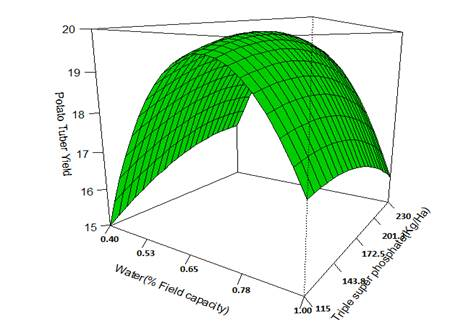Figure 2: Potato tuber yield as a function of Water and Phosphorus as at 124.7Kg/Ha of Nitrogen mineral nutrient

Figure 2 denotes the surface plot of the potato yield as a function of Water and Phosphorus at Nitrogen of 124.75Kg/Ha. This figure show that Water and Phosphorus nutrient have a direct effect on the yield of potato up to a certain level and then yield of potato decreased with increasing the water and Phosphorus nutrient. An increase of water and Phosphorus nutrient, up to a maximum of 70.04% field capacity and 191.04Kg/Ha of Phosphorus nutrient supplied as Triple super phosphate respectively would give a maximum potato tuber yield of 19.36Kg of potato tuber per plot of 1.8meters by 2.25meters.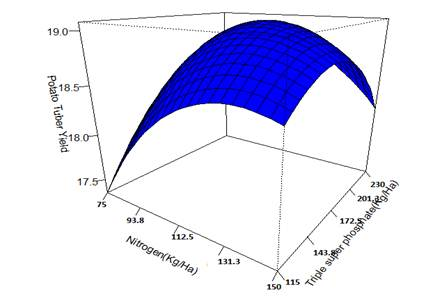Figure 3: Potato tuber yield as a function of Nitrogen and Phosphorus as at 70.04% field capacity

Figure 3 denotes the surface plot of the potato yield as a function of Nitrogen and Phosphorus at 70.04% water field capacity. It seems that the effect of Nitrogen and Phosphorus is significant and the value of interaction coefficient (p<0.05) demonstrate this fact.

3.3. Optimization of Production Conditions

In order to optimize production condition, the first partial derivatives of the regression model were equated to zero according to x1, x2 and x3 respectively. The result was calculated as shown in Table 4.

Table 4: Optimization of production conditions

 Variables Description Optimal values X1 Water 70.04% Field capacity X2 Nitrogen 124.75Kg/Ha X3 Phosphorus 191.04Kg/HaPotato tuber yield( Kg/Plot) 19.364Kg/Plot

Under such condition, the potato tuber yield was predicted to be 19.14Kg/plot (1.8meters by 2.25 meters). The experimental work at this condition was performed due to maximum experimental yield. In this work, highest potato tuber yield at 70.04% water saturation, 124.75Kg/Ha of Nitrogen supplied as urea and 191.04Kg/Ha phosphorus supplied as triple super phosphate (TSP), containing 46% P2O5, at planting time is obtained 19.36Kg/plot.

4. Conclusions and Recommendation

The study was aimed at determining the optimal operating conditions for potato production. Process optimization was accomplished by applying factorial design and response surface methodology. This study clearly shows that response surface methodology was a suitable method to optimize the operating conditions in order to maximize the potato tuber yield. Graphical response surfaces were used to locate the optimum point. The potato production has a negative quadratic behaviour by water, Nitrogen and phosphorus. It was predicted that the optimum operating condition within the experimental range would be 70.04% water saturation, 124.75Kg/Ha of Nitrogen supplied as urea and 191.04Kg/Ha phosphorus supplied as triple super phosphate (TSP). At the optimum condition one can reach to a potato tuber yield of 19.36Kg/plot of 1.8meters by 2.25 meters. Increased productivity of potatoes can improve the livelihood of smallholder potato farmers in Kenya and safe the farmers extra cost of input. Finally, i hope that the approach applied in this study of potatoes can be useful for research on other commodities, leading to a better understanding of overall crop production.

Acknowledgment

I wish to acknowledge with thanks Dr. K.Gathungu from Chuka University and his team for their support and willingness to provide information (data) needed for this study. In addition, I am indedted to Prof. Kosgei (Moi Univesity) who introduced me to the subject of design of Experiment and in particular response surface methodology.

References

1. Alem G.(1993). Evaluation of tillage practices for soil moisture conservation and maize production in dryland Ethiopia. Agricultural Mechanization in Asia, Africa and Latin America. ;24(3):9–13.
2. D. K. Muriithi, J. Kihoro and A. Kihoro.(2012). Ordinal logistic regression versus multiple binary logistic regression model for predicting student loan allocation, Journal of Agriculture, Science and Technology 14(1).
3. D. K. Muriithi, G. G. Njoroge, E. W. Njoroge and O. Mark, (2013). Classification of higher education loans using multinomial logistic regression model, Journal of Mathematical Sciences: Advances and Applications Vol. 22, PP. 1-17.
4. D .K. Muriithi,A. N. Ngeretha, R. G. Muriungi And E. W. Njoroge.(2014). Analysis of the Fluctuation of Gross Domestic Product In Kenya Using Autoregressive Integrated Moving Average Model. Journal of Statistics: Advances in Theory and Applications.Vol.11, No.1, 2014, PP31-43
5. G.E.P. Box, W.G. Hunter, J.S. Hunter,(1978). Statistics for Experimenters: An Introduction to Design, Data Analysis and Model Building, John Wiley, New York.
6. Giovanilton F. S, Fernando L. C, Andrea L.O.F.(2011). Application of response surface methodology for optimization of biodiesel production by transesterification of soybean oil with ethanol. Fuel Processing Technology, Vol 92: pp 407-413
7. K.Gathungu.G, N.Aguyoh.J and K.Isutsa Dorcas.(2014). Optimizing Seed Potato (Solanum tuberosum L.) Tuber Yield and Size Distribution through Integrated Irrigation Water, Nitrogen and Phosphorus Mineral Nutrient Application. American Journal of experimental Agriculture, Vol 4(3): pp 349-361
8. Khuri, A. I. and Cornell, J. A.,(1987). Response Surfaces: Designs and Analyses, Marcel Dekker, New York, NY.
9. Montgomery, D. C. (2001). Design and Analysis of Experiments, JohnWiley and Sons, New York, NY.
10. Myers, R. H., Montgomery, D. C., Vining, G. G., Borror, C. M. and Kowalski, S. M. (2004). "Response Surface Methodology: A Retrospective and Literature Survey," J. Qual. Technol., Vol. 36, pp. 5377.
11. Koksoy, O., "Dual Response Optimization: The DesirabilityApproach," Int. J. Ind. Eng.-Theory, Vol. 12,
12. Chih-Wei Tsai, Lee-Ing Tong and Chung-Ho Wang.(2010). Optimization of Multiple Responses Using Data Envelopment Analysis and Response Surface Methodology, Tamkang Journal of Science and Engineering, Vol. 13, No. 2, pp.197-203

 Contents 1. 2. 2.1. 2.2. 3. 3.1. 3.2. 3.3. 4.
Article ToolsAbstractPDF(267K)